# 8 6 Proportions Similar Triangles Geometry Mrs Spitz

• Slides: 20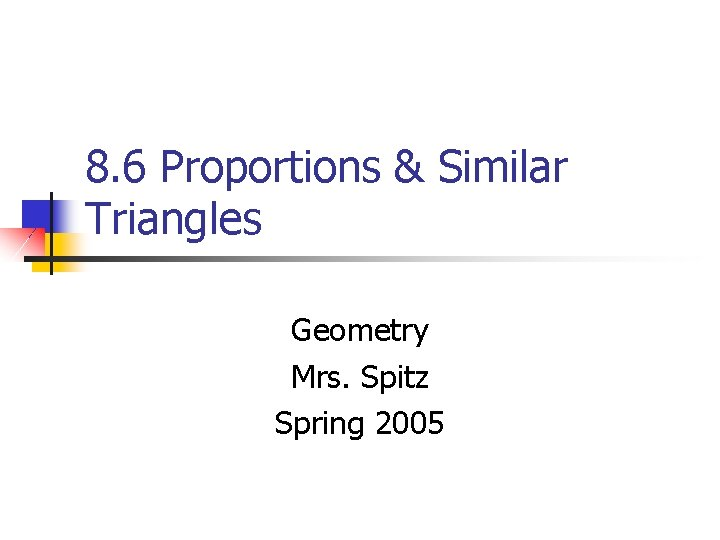8. 6 Proportions & Similar Triangles Geometry Mrs. Spitz Spring 2005Objectives/Assignments n n n Use proportionality theorems to calculate segment lengths. To solve real-life problems, such as determining the dimensions of a piece of land. Assignment: pp. 502 -503 #1 -30Use Proportionality Theorems n In this lesson, you will study four proportionality theorems. Similar triangles are used to prove each theorem.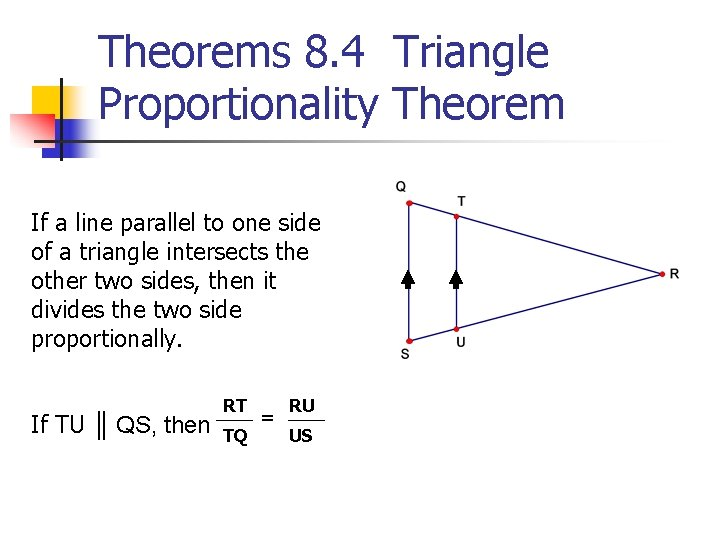Theorems 8. 4 Triangle Proportionality Theorem If a line parallel to one side of a triangle intersects the other two sides, then it divides the two side proportionally. If TU ║ QS, then RT TQ = RU US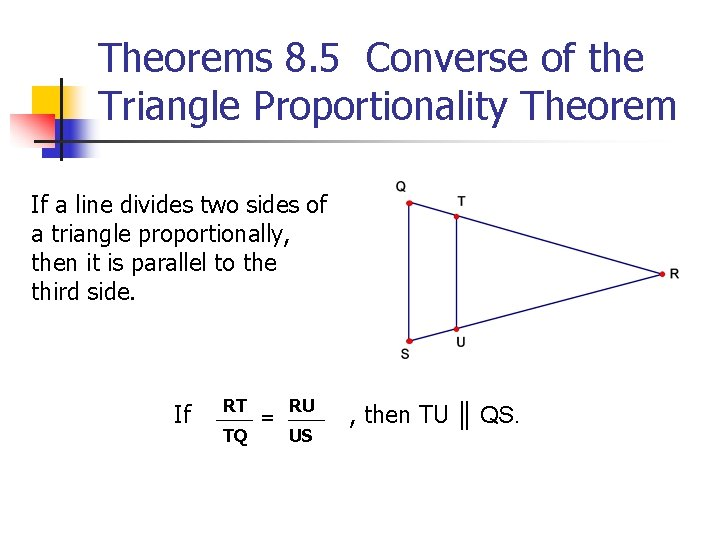Theorems 8. 5 Converse of the Triangle Proportionality Theorem If a line divides two sides of a triangle proportionally, then it is parallel to the third side. If RT TQ = RU US , then TU ║ QS.Ex. 1: Finding the length of a segment n In the diagram AB ║ ED, BD = 8, DC = 4, and AE = 12. What is the length of EC?Step: DC = EC BD AE 4 EC = 8 12 4(12) = EC 8 6 = EC ØSo, the length of EC is 6. Reason Triangle Proportionality Thm. Substitute Multiply each side by 12. Simplify.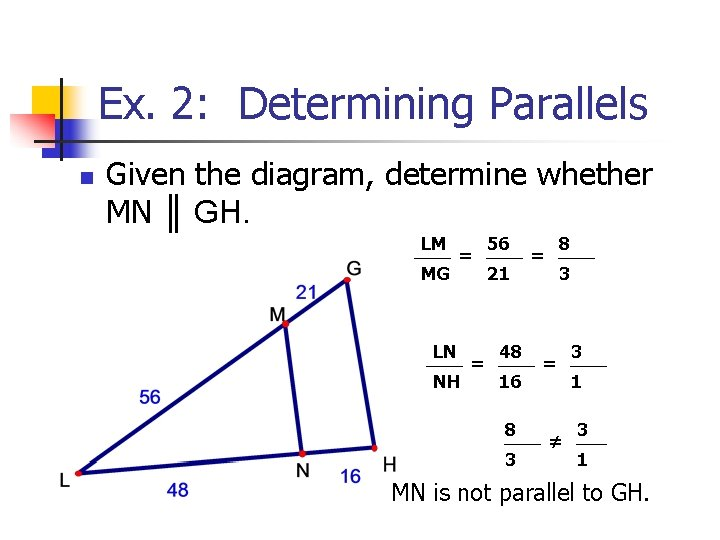Ex. 2: Determining Parallels n Given the diagram, determine whether MN ║ GH. LM MG = LN NH = 56 21 48 16 8 3 8 = 3 = ≠ 3 1 MN is not parallel to GH.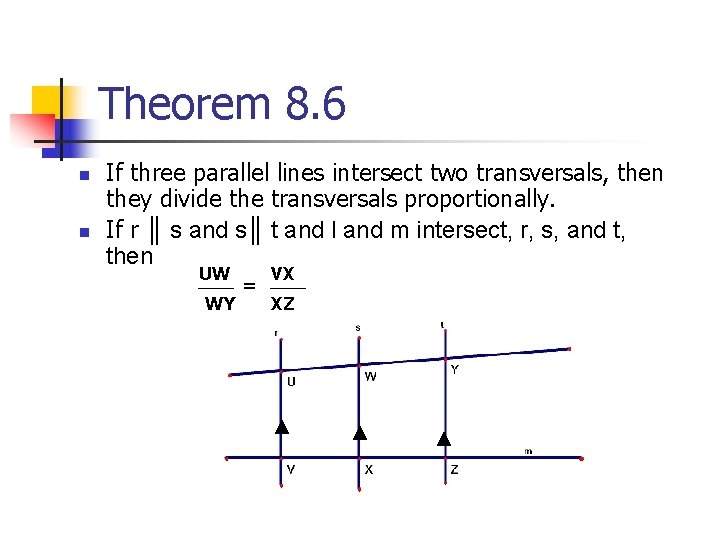Theorem 8. 6 n n If three parallel lines intersect two transversals, then they divide the transversals proportionally. If r ║ s and s║ t and l and m intersect, r, s, and t, then UW WY = VX XZTheorem 8. 7 n n If a ray bisects an angle of a triangle, then it divides the opposite side into segments whose lengths are proportional to the lengths of the other two sides. AD CA = If CD bisects ACB, then DB CB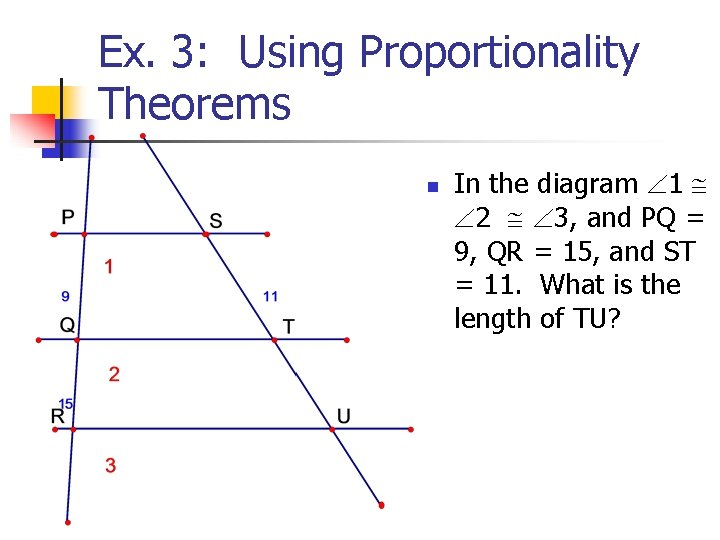Ex. 3: Using Proportionality Theorems n In the diagram 1 2 3, and PQ = 9, QR = 15, and ST = 11. What is the length of TU?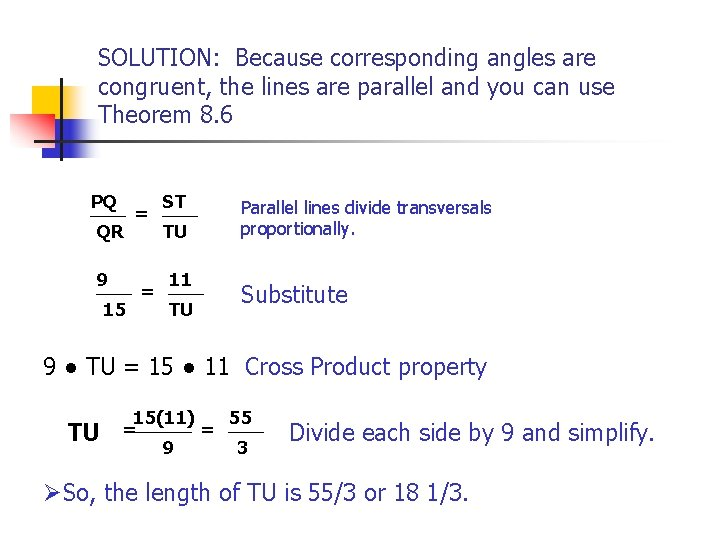SOLUTION: Because corresponding angles are congruent, the lines are parallel and you can use Theorem 8. 6 PQ QR 9 15 = = ST TU 11 TU Parallel lines divide transversals proportionally. Substitute 9 ● TU = 15 ● 11 Cross Product property TU 15(11) 55 = = 9 3 Divide each side by 9 and simplify. ØSo, the length of TU is 55/3 or 18 1/3.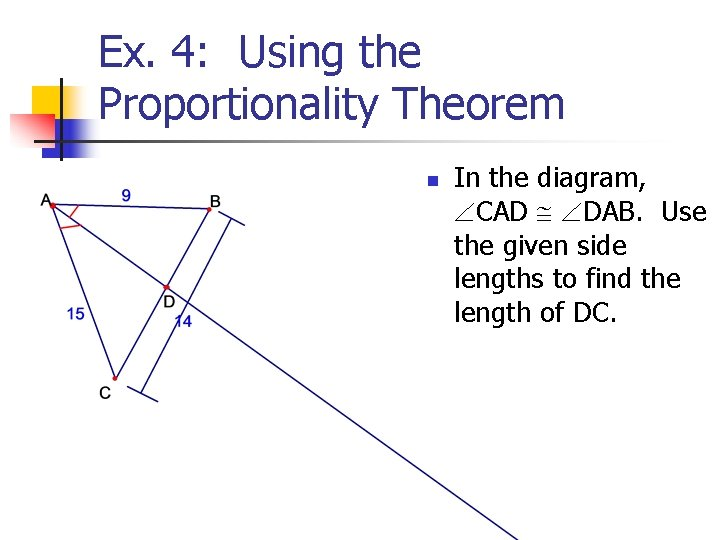Ex. 4: Using the Proportionality Theorem n In the diagram, CAD DAB. Use the given side lengths to find the length of DC.Solution: Since AD is an angle bisector of CAB, you can apply Theorem 8. 7. Let x = DC. Then BD = 14 – x. AB AC 9 15 = = BD DC 14 -X X Apply Thm. 8. 7 Substitute.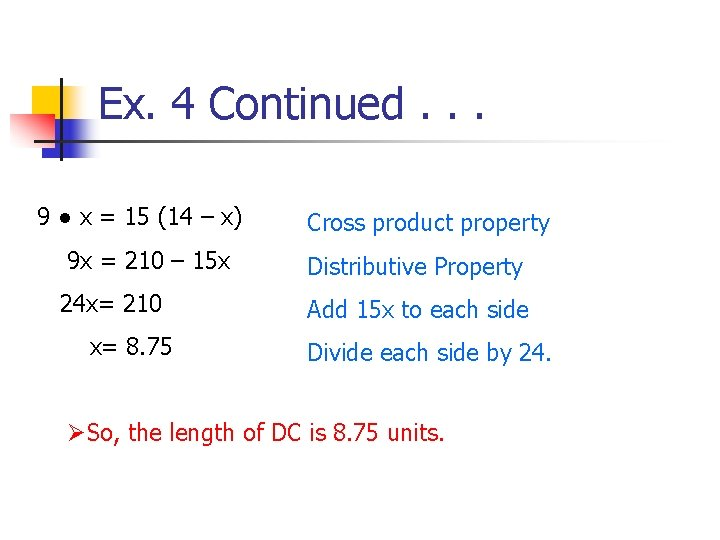Ex. 4 Continued. . . 9 ● x = 15 (14 – x) 9 x = 210 – 15 x 24 x= 210 x= 8. 75 Cross product property Distributive Property Add 15 x to each side Divide each side by 24. ØSo, the length of DC is 8. 75 units.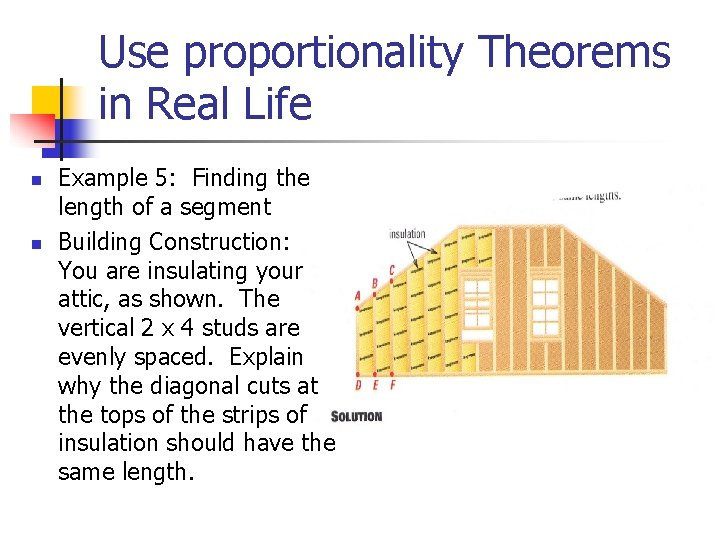Use proportionality Theorems in Real Life n n Example 5: Finding the length of a segment Building Construction: You are insulating your attic, as shown. The vertical 2 x 4 studs are evenly spaced. Explain why the diagonal cuts at the tops of the strips of insulation should have the same length.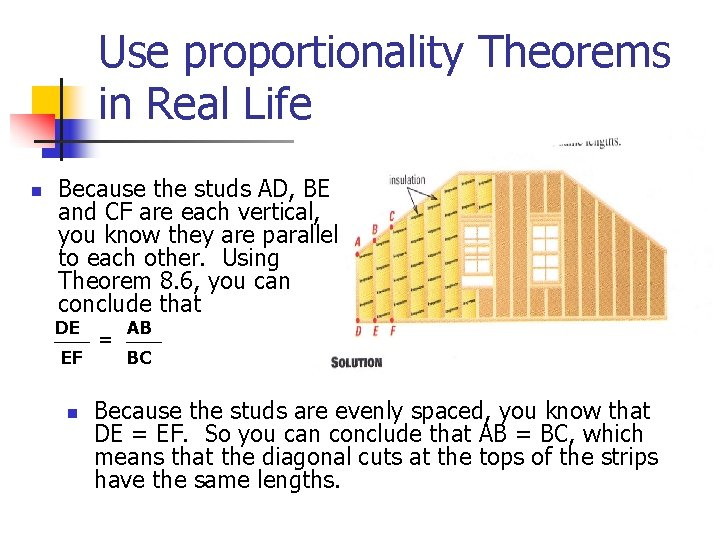Use proportionality Theorems in Real Life n Because the studs AD, BE and CF are each vertical, you know they are parallel to each other. Using Theorem 8. 6, you can conclude that DE EF n = AB BC Because the studs are evenly spaced, you know that DE = EF. So you can conclude that AB = BC, which means that the diagonal cuts at the tops of the strips have the same lengths.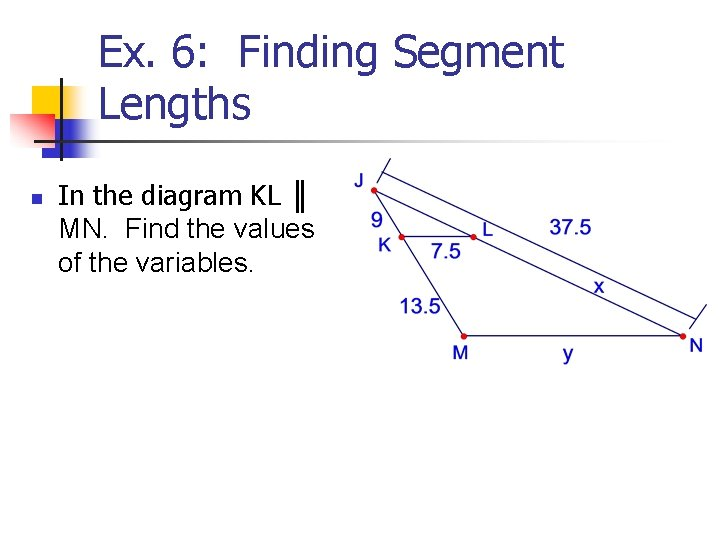Ex. 6: Finding Segment Lengths n In the diagram KL ║ MN. Find the values of the variables.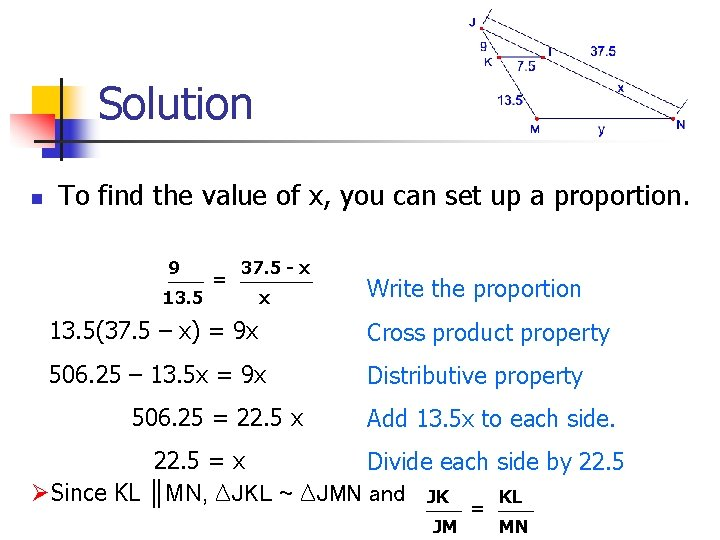Solution n To find the value of x, you can set up a proportion. 9 13. 5 = 37. 5 - x x Write the proportion 13. 5(37. 5 – x) = 9 x Cross product property 506. 25 – 13. 5 x = 9 x Distributive property 506. 25 = 22. 5 x Add 13. 5 x to each side. 22. 5 = x Divide each side by 22. 5 ØSince KL ║MN, ∆JKL ~ ∆JMN and JK KL JM = MN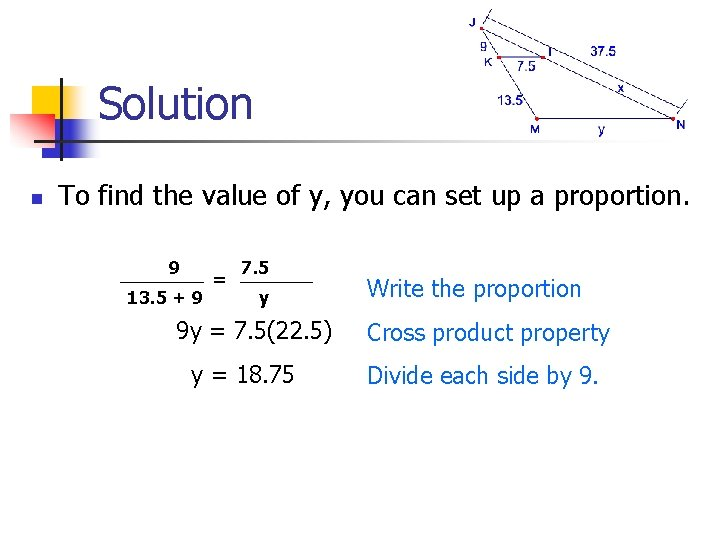Solution n To find the value of y, you can set up a proportion. 9 13. 5 + 9 = 7. 5 y 9 y = 7. 5(22. 5) y = 18. 75 Write the proportion Cross product property Divide each side by 9.